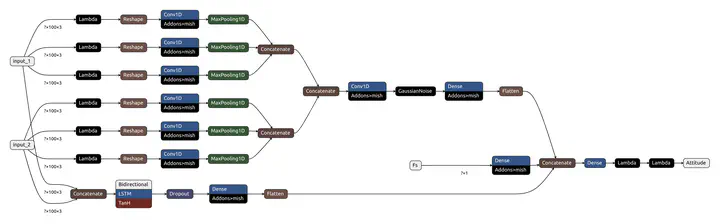# Deep Learning based Inertial Attitude EstimationThis project aimed for training and evaluating a deep learning model for predicting quaternion-based orientations using inertial measurement unit (IMU) sensor data. The model is trained using a combination of accelerometer, gyroscope, and magnetometer readings from the IMU sensors.# End-to-End-Deep-Learning-Framework-for-Real-Time-Inertial-Attitude-Estimation-using-6DoF-IMU

Code repo of paper Generalizable end-to-end deep learning frameworks for real-time attitude estimation using 6DoF inertial measurement units

# IMU Quaternion Prediction

This repository contains code for training and evaluating a deep learning model for predicting quaternion orientations using inertial measurement unit (IMU) sensor data. The model is trained using a combination of accelerometer, gyroscope, and magnetometer readings from the IMU sensors.

## Introduction

Inertial measurement units (IMUs) are commonly used in various applications, such as robotics, virtual reality, and motion tracking. They consist of sensors, including accelerometers, gyroscopes, and magnetometers, that provide measurements of linear acceleration, angular velocity, and magnetic field orientation, respectively. Quaternion representations are often used to represent the orientation of an IMU sensor due to their advantages over other representations.

The goal of this project is to develop a deep learning model that can accurately predict the orientation of an IMU sensor using the sensor’s raw data. The model takes as input the accelerometer, gyroscope, and magnetometer readings and outputs a quaternion representing the sensor’s orientation in 3D space. Accurate quaternion prediction is crucial for applications that rely on precise orientation estimation.

## Requirements

To run the code in this repository, you need the following dependencies:

• Python 3.x
• TensorFlow 2.x
• Keras
• NumPy
• Pandas
• Matplotlib
• scikit-learn

These dependencies can be easily installed using pip or conda.

## Installation

1. Clone this repository to your local machine using the following command:
git clone https://github.com/your-username/imu-quaternion-prediction.git

2. Change into the project directory:
cd imu-quaternion-prediction

3. Install the required dependencies using pip:
pip install -r requirements.txt


## Usage

The code in this repository is organized into several modules, each responsible for a specific task. Here is an overview of the main modules and their functionality:

• model.py: Contains the definition of the deep learning model used for quaternion prediction. It utilizes convolutional and recurrent neural network layers to learn spatial and temporal patterns from the sensor data.
• dataset_loader.py: Implements functions for loading and preprocessing the IMU sensor data. It handles reading data from different sources, merging the data into a single dataset, and splitting it into training and testing sets. It also performs windowing and normalization of the sensor data.
• learning.py: Includes functions for training and evaluating the model. It compiles the model with an appropriate optimizer and loss function, sets up callbacks for early stopping, learning rate scheduling, model checkpointing, and tensorboard logging. It also trains the model using the training dataset and evaluates its performance on the testing dataset.
• util.py: Provides utility functions used throughout the project, such as functions for computing quaternion error angles and visualizing sensor data.

To run the code, you can use the train.py script. This script loads the IMU sensor data, preprocesses it, trains the model, and evaluates its performance. You can customize the hyperparameters and settings in the script to suit your needs.

To train the model, run the following command:

python train.py


## Data Preprocessing

The dataset_loader.py module provides functions for loading and preprocessing the IMU sensor data. The data preprocessing

steps include reading the sensor data from CSV files, merging the data from multiple sensors into a single dataset, splitting the data into training and testing sets, windowing the data, and normalizing the sensor readings. These preprocessing steps are essential to prepare the data for training the model.

## Model Training

The model.py module contains the definition of the deep learning model used for quaternion prediction. The model architecture consists of convolutional and recurrent neural network layers, which enable the model to learn spatial and temporal patterns from the sensor data. The model is compiled with an appropriate optimizer and loss function for training.

During training, the model is fed with batches of preprocessed sensor data. The model learns to map the input sensor readings to quaternion orientations by minimizing the specified loss function. The training process can be customized using various hyperparameters, such as learning rate, batch size, and number of epochs.

## Model Evaluation

After training, the model’s performance is evaluated using the testing dataset. The learning.py module includes functions for evaluating the model’s performance by computing various metrics, such as mean absolute error (MAE), root mean squared error (RMSE), and mean error angle (MEA). These metrics provide insights into how well the model is performing and help you understand its strengths and weaknesses.

## Results

The results directory contains the trained models and evaluation results obtained during the experiments. You can find the best-performing models and their corresponding evaluation metrics in this directory. The evaluation results include metrics such as MAE, RMSE, and MEA, as well as visualizations of the predicted quaternion orientations compared to the ground truth values.

## Contributing

Contributions to this repository are welcome. If you have any suggestions, bug fixes, or improvements, feel free to submit a pull request. Please provide clear and detailed information about the changes you have made.

## Citation

If you make use of this work, please cite

@software{Asgharpoor_Golroudbari_End-to-End-Deep-Learning-Framework-for-Real-Time-Inertial-Attitude-Estimation-using-6DoF-IMU_2023,
author = {Asgharpoor Golroudbari, Arman and Sabour, Mohammad Hossein},
doi = {10.5281/zenodo.7850047},
month = apr,
title = {{End-to-End-Deep-Learning-Framework-for-Real-Time-Inertial-Attitude-Estimation-using-6DoF-IMU}},
url = {https://github.com/Armanasq/End-to-End-Deep-Learning-Framework-for-Real-Time-Inertial-Attitude-Estimation-using-6DoF-IMU},
version = {1.0.0},
year = {2023}
}

@article{GOLROUDBARI2023113105,
title = {Generalizable end-to-end deep learning frameworks for real-time attitude estimation using 6DoF inertial measurement units},
journal = {Measurement},
pages = {113105},
year = {2023},
issn = {0263-2241},
doi = {https://doi.org/10.1016/j.measurement.2023.113105},
url = {https://www.sciencedirect.com/science/article/pii/S0263224123006693},
author = {Arman Asgharpoor Golroudbari and Mohammad Hossein Sabour},
keywords = {Deep learning, Navigation, Inertial sensors, Intelligent filter, Sensor fusion, Long-short term memory, Convolutional neural network}